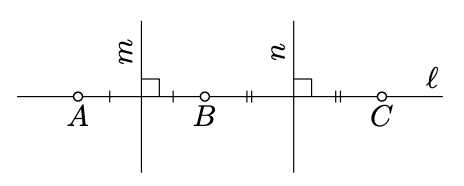$$\newcommand{\id}{\mathrm{id}}$$ $$\newcommand{\Span}{\mathrm{span}}$$ $$\newcommand{\kernel}{\mathrm{null}\,}$$ $$\newcommand{\range}{\mathrm{range}\,}$$ $$\newcommand{\RealPart}{\mathrm{Re}}$$ $$\newcommand{\ImaginaryPart}{\mathrm{Im}}$$ $$\newcommand{\Argument}{\mathrm{Arg}}$$ $$\newcommand{\norm}{\| #1 \|}$$ $$\newcommand{\inner}{\langle #1, #2 \rangle}$$ $$\newcommand{\Span}{\mathrm{span}}$$

# 5.6: Circles

$$\newcommand{\vecs}{\overset { \rightharpoonup} {\mathbf{#1}} }$$ $$\newcommand{\vecd}{\overset{-\!-\!\rightharpoonup}{\vphantom{a}\smash {#1}}}$$$$\newcommand{\id}{\mathrm{id}}$$ $$\newcommand{\Span}{\mathrm{span}}$$ $$\newcommand{\kernel}{\mathrm{null}\,}$$ $$\newcommand{\range}{\mathrm{range}\,}$$ $$\newcommand{\RealPart}{\mathrm{Re}}$$ $$\newcommand{\ImaginaryPart}{\mathrm{Im}}$$ $$\newcommand{\Argument}{\mathrm{Arg}}$$ $$\newcommand{\norm}{\| #1 \|}$$ $$\newcommand{\inner}{\langle #1, #2 \rangle}$$ $$\newcommand{\Span}{\mathrm{span}}$$ $$\newcommand{\id}{\mathrm{id}}$$ $$\newcommand{\Span}{\mathrm{span}}$$ $$\newcommand{\kernel}{\mathrm{null}\,}$$ $$\newcommand{\range}{\mathrm{range}\,}$$ $$\newcommand{\RealPart}{\mathrm{Re}}$$ $$\newcommand{\ImaginaryPart}{\mathrm{Im}}$$ $$\newcommand{\Argument}{\mathrm{Arg}}$$ $$\newcommand{\norm}{\| #1 \|}$$ $$\newcommand{\inner}{\langle #1, #2 \rangle}$$ $$\newcommand{\Span}{\mathrm{span}}$$

Recall that a circle with radius $$r$$ and center $$O$$ is the set of all points on distance $$r$$ from $$O$$. We say that a point $$P$$ lies inside of the circle if $$OP < r$$; if $$OP > r$$, we say that $$P$$ lies outside of the circle.

Exercise $$\PageIndex{1}$$

Let $$\Gamma$$ be a circle and $$P \not\in \Gamma$$. Assume a line $$\ell$$ is passing thru the point $$P$$ and intersects $$\Gamma$$ at two distinct points, $$X$$ and $$Y$$. Show that $$P$$ is inside $$\Gamma$$ if and only if $$P$$ lies between $$X$$ and $$Y$$.

Hint

Let $$O$$ be the center of the circle. Note that we can assume that $$O \ne P$$.

Assume $$P$$ lies between $$X$$ and $$Y$$. By Exercise 5.1.1, we can assume that $$OPX$$ is right or obtuse. By Exercise 5.5.1, $$OP < OX$$; that is, $$P$$ lies inside $$\Gamma$$.

If $$P$$ does not lie between $$X$$ and $$Y$$, we can assume that $$X$$ lies between $$P$$ and $$Y$$. Since $$OX = OY$$, Exercise 5.5.1 implies that $$\angle OXY$$ is acute. Therefore, $$\angle OXP$$ is obtuse. Applying Exercise 5.5.1 again we get that $$OP > OX$$l that is, $$P$$ lies outside $$\Gamma$$.

A segment between two points on a circle is called a chord of the circle. A chord passing thru the center of the circle is called its diameter.

Exercise $$\PageIndex{2}$$

Assume two distinct circles $$\Gamma$$ and $$\Gamma'$$ have a common chord $$[AB]$$. Show that the line between centers of $$\Gamma$$ and $$\Gamma'$$ forms a perpendicular bisector to $$[AB]$$.

Hint

Apply Theorem 5.2.1.

Lemma $$\PageIndex{1}$$

A line and a circle can have at most two points of intersection.

ProofAssume $$A, B$$, and $$C$$ are distinct points that lie on a line $$\ell$$ and a circle $$\Gamma$$ with the center $$O$$. Then $$OA = OB = OC$$; in particular, $$O$$ lies on the perpendicular bisectors $$m$$ and $$n$$ to $$[AB]$$ and $$[BC]$$ respectively. Note that the midpoints of $$[AB]$$ and $$[BC]$$ are distinct. Therefore, $$m$$ and $$n$$ are distinct. The contradicts the uniqueness of the perpendicular (Theorem 5.3.1).

Exercise $$\PageIndex{3}$$

Show that two distinct circles can have at most two points of intersection.

Hint

Use Exercise $$\PageIndex{2}$$ and Theorem 5.3.1.

In consequence of the above lemma, a line $$\ell$$ and a circle $$\Gamma$$ might have 2, 1 or 0 points of intersections. In the first case the line is called secant line, in the second case it is tangent line; if $$P$$ is the only point of intersection of $$\ell$$ and $$\Gamma$$, we say that $$\ell$$ is tangent to $$\Gamma$$ at $$P$$.

Similarly, according Exercise $$\PageIndex{3}$$, two distinct circles might have 2, 1 or 0 points of intersections. If $$P$$ is the only point of intersection of circles $$\Gamma$$ and $$\Gamma'$$, we say that $$\Gamma$$ is tangent to $$\Gamma$$ at $$P$$; we also assume that circle is tangent to it self at any of its points.

Lemma $$\PageIndex{2}$$

Let $$\ell$$ be a line and $$\Gamma$$ be a circle with the center $$O$$. Assume $$P$$ is a common point of $$\ell$$ and $$\Gamma$$. Then $$\ell$$ is tangent to $$\Gamma$$ at $$P$$ if and only if $$(PQ) \perp \ell$$.

Proof

Let $$Q$$ be the foot point of $$O$$ on $$\ell$$.

Assume $$P \ne Q$$. Let $$P'$$ be the reflection of $$P$$ across $$(OQ)$$. Note that $$P' \in \ell$$ and $$(OQ)$$ is the perpendicular bisector of $$[PP']$$. Therefore, $$OP = OP'$$. Hence $$P, P' \in \Gamma \cap \ell$$; that is, $$\ell$$ is secant to $$\Gamma$$.

If $$P = Q$$, then according to Lemma 5.5.1, $$OP < OX$$ for any point $$X \in \ell$$ distinct from $$P$$. Hence $$P$$ is the only point in the intersection $$\Gamma \cap \ell$$; that is, $$\ell$$ is tangent to $$\Gamma$$ at $$P$$.

Exercise $$\PageIndex{4}$$

Let $$\Gamma$$ and $$\Gamma'$$ be two distinct circles with centers at $$O$$ and $$O'$$ respectively. Assume $$\Gamma$$ meets $$\Gamma'$$ at point $$P$$. Show that $$\Gamma$$ is tangent to $$\Gamma'$$ if and only if $$O$$, $$O'$$, and $$P$$ lie on one line.

Hint

Let $$P'$$ be the reflection of $$P$$ across $$(OO')$$. Note that $$P'$$ lies on both circles and $$P' \ne P$$ if and only if $$P \not\in (OO')$$.

Exercise $$\PageIndex{5}$$

Let $$\Gamma$$ and $$\Gamma'$$ be two distinct circles with centers at $$O$$ and $$O'$$ and radiuses $$r$$ and $$r'$$. Show that $$\Gamma$$ is tangent to $$\Gamma'$$ if and only if

$$OO' = r + r'$$       or       $$OO' = |r - r'|$$.

Hint

Apply Exercise $$\PageIndex{4}$$

Exercise $$\PageIndex{6}$$

Assume three circles have two points in common. Prove that their centers lie on one line.Hint

Let $$A$$ and $$B$$ be the points of intersection. Note that the centers lie on the perpendicular bisector of the segment $$[AB]$$.